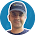## Pages

SUBSCRIBE HERE TO GET POST UPDATES VIA EMAIL :

Delivered by FeedBurner

# Interview Questions on Java For Selenium

Part 6

26 : What is local variable in java?
Answer : Local variable is declared inside method or constructor and it is limited for that method or constructor only. View more detail on local variable in java.

Local Variable Example :
``````public class JavaVariable {

public void Calc() {
// Local Variables.
int sum;
int item1 = 5;
int item2 = 7;
sum = item1 + item2;
System.out.println("Sum is : " + sum);
}

public static void main(String args[]) {
JavaVariable j = new JavaVariable();
j.Calc();
}
}``````

27 : What is a Instance Variable in java?
Answer : Instance Variable is declared parallel to method or constructor in class. It is visible for all methods and constructors of that class. View more detail on instance variable in java.

Instance Variable Example :
``````public class JavaVariable {
//Instance Variable.
int sum;

public void Calc() {
// Local Variables.
int item1 = 5;
int item2 = 7;
sum = item1 + item2;
System.out.println("Sum is : " + sum);
}

public static void main(String args[]) {
JavaVariable j = new JavaVariable();
j.Calc();
}``````

28 : What is a Class Variable in java?
Answer : Class variable is declared with static keyword in class parallel to methods and constructor. Class variable is initialized only once at the start of execution and destroyed on end of program. Class variable is also known as static variable. View more detail on class variable in java.

Class Variable Example :
``````public class JavaVariable {
//Class Variable
//Instance Variable.
int sum;

public void Calc() {
// Local Variables.
int item1 = 5;
int item2 = 7;
sum = item1 + item2;
System.out.println(sumType + " Sum is : " + sum);
}

public static void main(String args[]) {
JavaVariable j = new JavaVariable();
j.Calc();
}``````

29 : What is the difference between instance variable and class variable?

Instance Variable :
• It is unique to each instance of the class.
• Declared without static modifier.
Static Variable :
• It is shared by all instances of the class.
• Declared with static modifier.
• Memory allocation is done at compile time, loaded at load time and they are initialized at class initialization time.
30. What is access modifier in java?
Answer : Access modifiers allows us to set access levels for variables, methods, classes and constructors in java. We can control access levels using access modifiers in java. View more details on different access modifiers in java.

1.2.